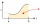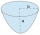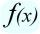# Integral - math problems

The integral is used for calculating the general area, the volume of the sum. Definite integral is actually the content area under the graph of the function at some certain interval . The integral symbol ∫ is derived from the letter S - sum.

#### Number of problems found: 5

• A particleA particle moves in a straight line so that its velocity (m/s) at time t seconds is given by v(t) = 3t2-4t-4, t>0. Initially the particle is 8 meters to the right of a fixed origin. After how many seconds is the particle at the origin?
• The parabolic segmentThe parabolic segment has a base a = 4 cm and a height v = 6 cm. Calculate the volume of the body that results from the rotation of this segment a) around its base b) around its axis.
• Cylinder horizontallyThe cylinder with a diameter of 3 m and a height/length of 15 m is laid horizontally. Water is poured into it, reaching a height of 60 cm below the axis of the cylinder. How many hectoliters of water is in the cylinder?
• Truncated coneCalculate the height of the rotating truncated cone with volume V = 794 cm3 and a base radii r1 = 9.9 cm and r2 = 9.8 cm.
• DerivationExists a function whose derivation is the same function?

We apologize, but in this category are not a lot of examples.
Do you have an exciting math question or word problem that you can't solve? Ask a question or post a math problem, and we can try to solve it.

We will send a solution to your e-mail address. Solved examples are also published here. Please enter the e-mail correctly and check whether you don't have a full mailbox.

Please do not submit problems from current active competitions such as Mathematical Olympiad, correspondence seminars etc...